# A Simple Arduino Menu With An LCD

I've created a simple menu with an LCD that controls what an LED does. The menu is easy to edit and adapt for various projects.

IntermediateProtip1 hour77,944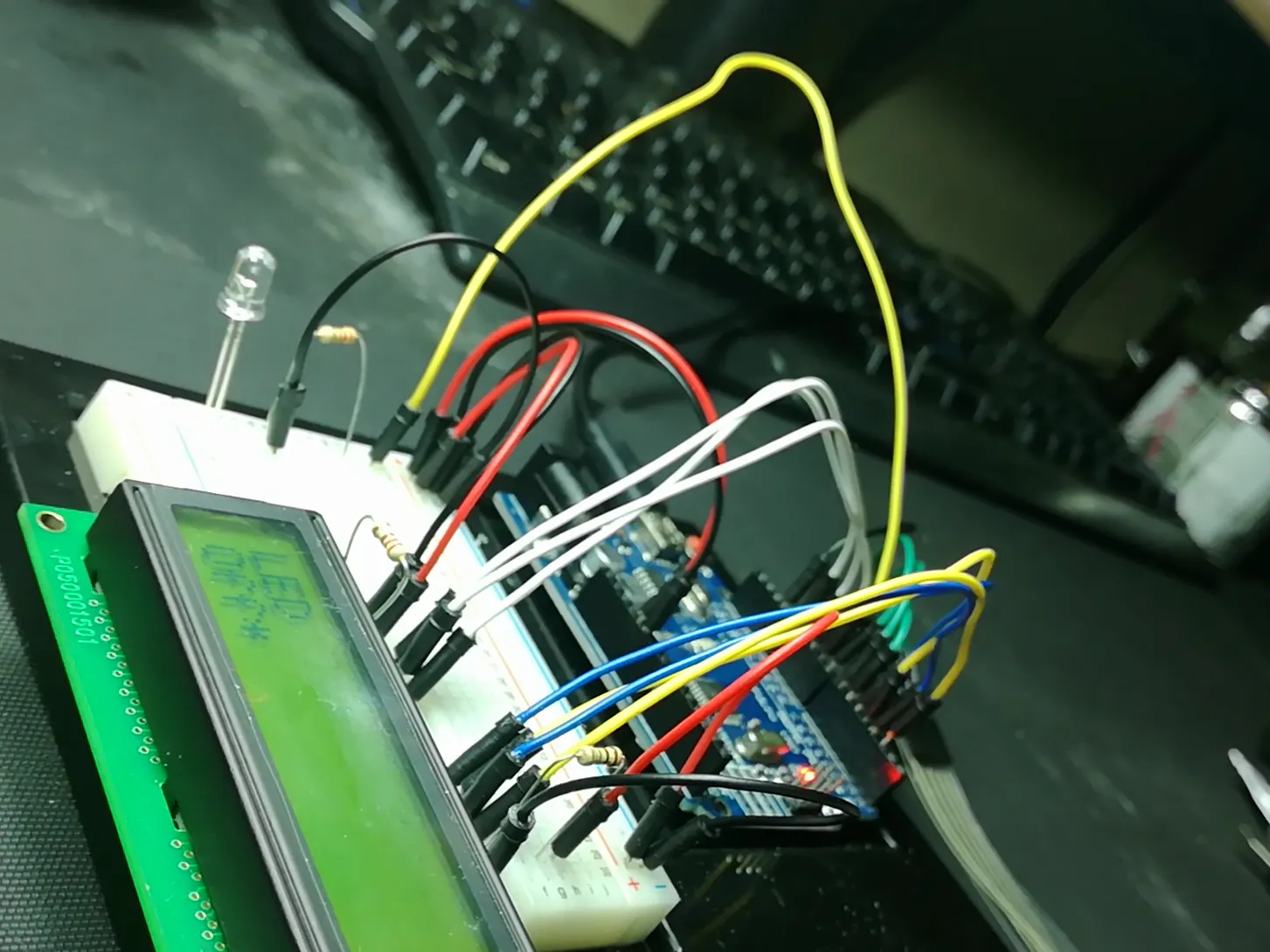## Things used in this project

### Hardware components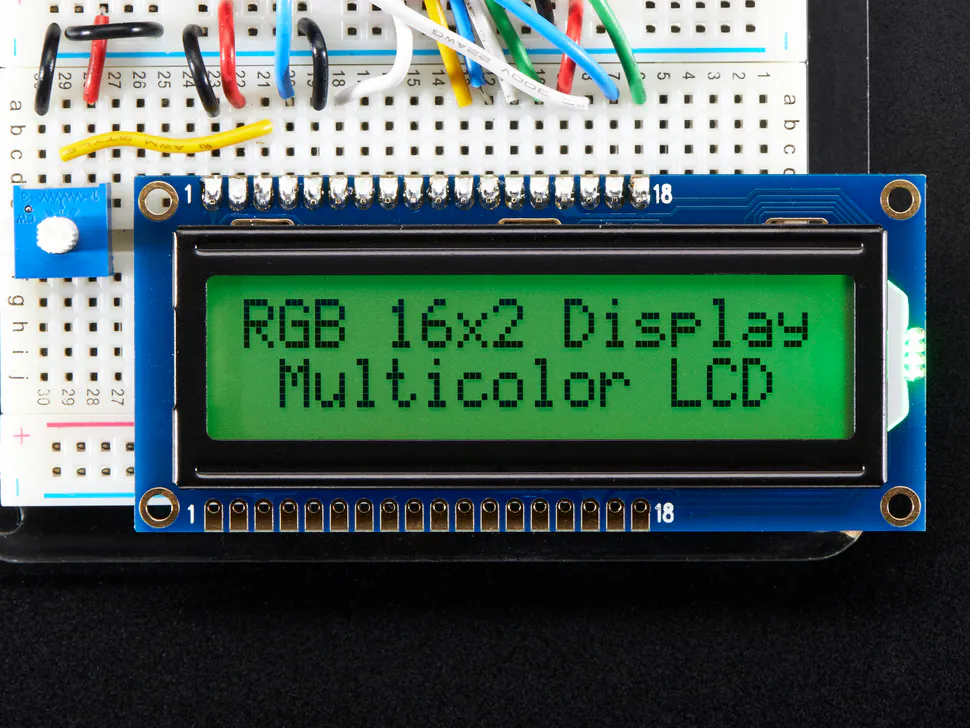Adafruit RGB Backlight LCD - 16x2
×1LED (generic)
×1Jumper wires (generic)
×1×1Arduino UNO & Genuino UNO
×1
 1 x 4 Digital Matrix Membrane Switch
×1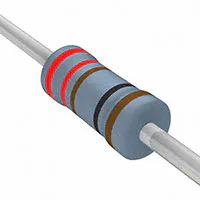Resistor 221 ohm
×2

## Schematics

### Schematics for the LCD Menu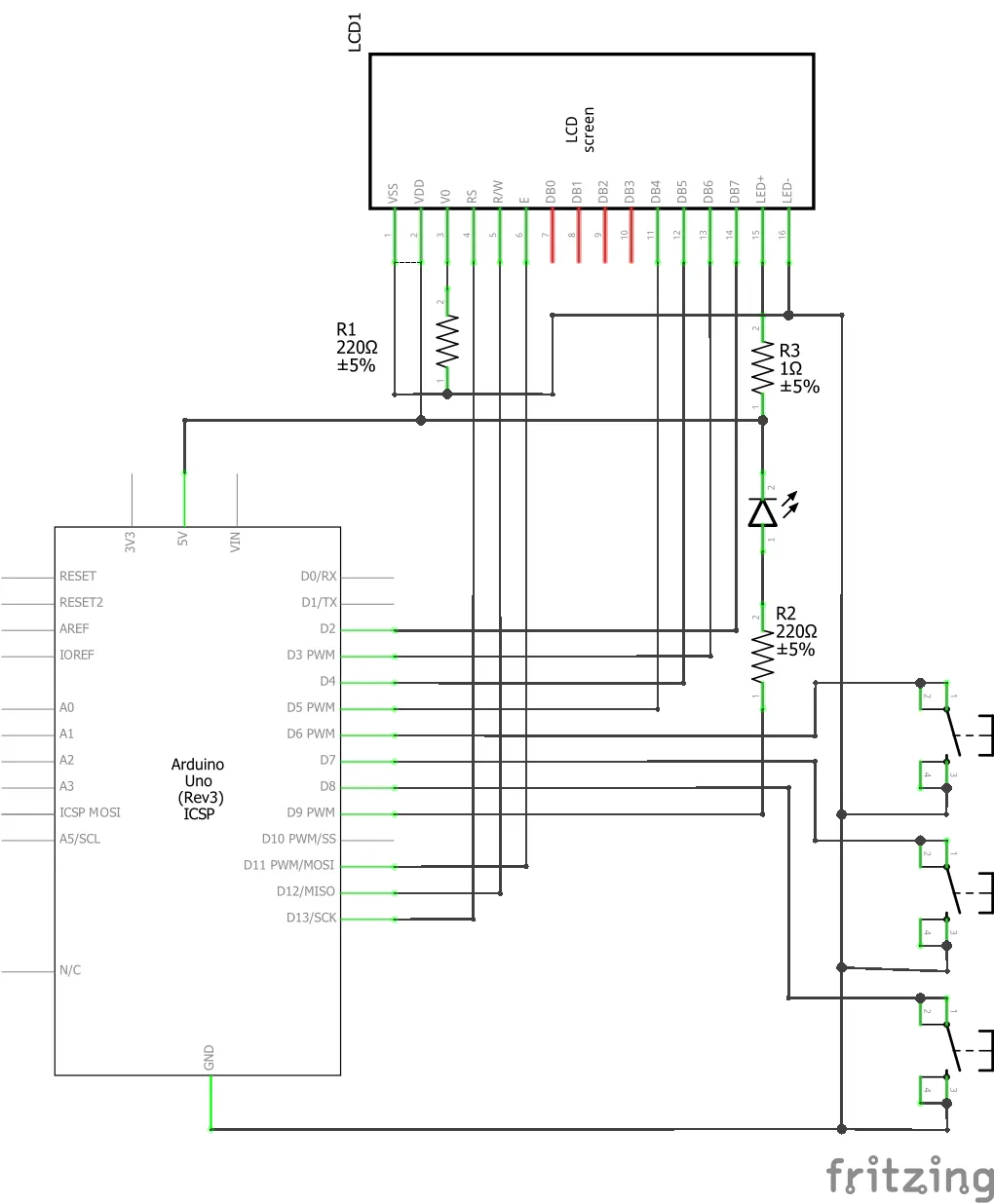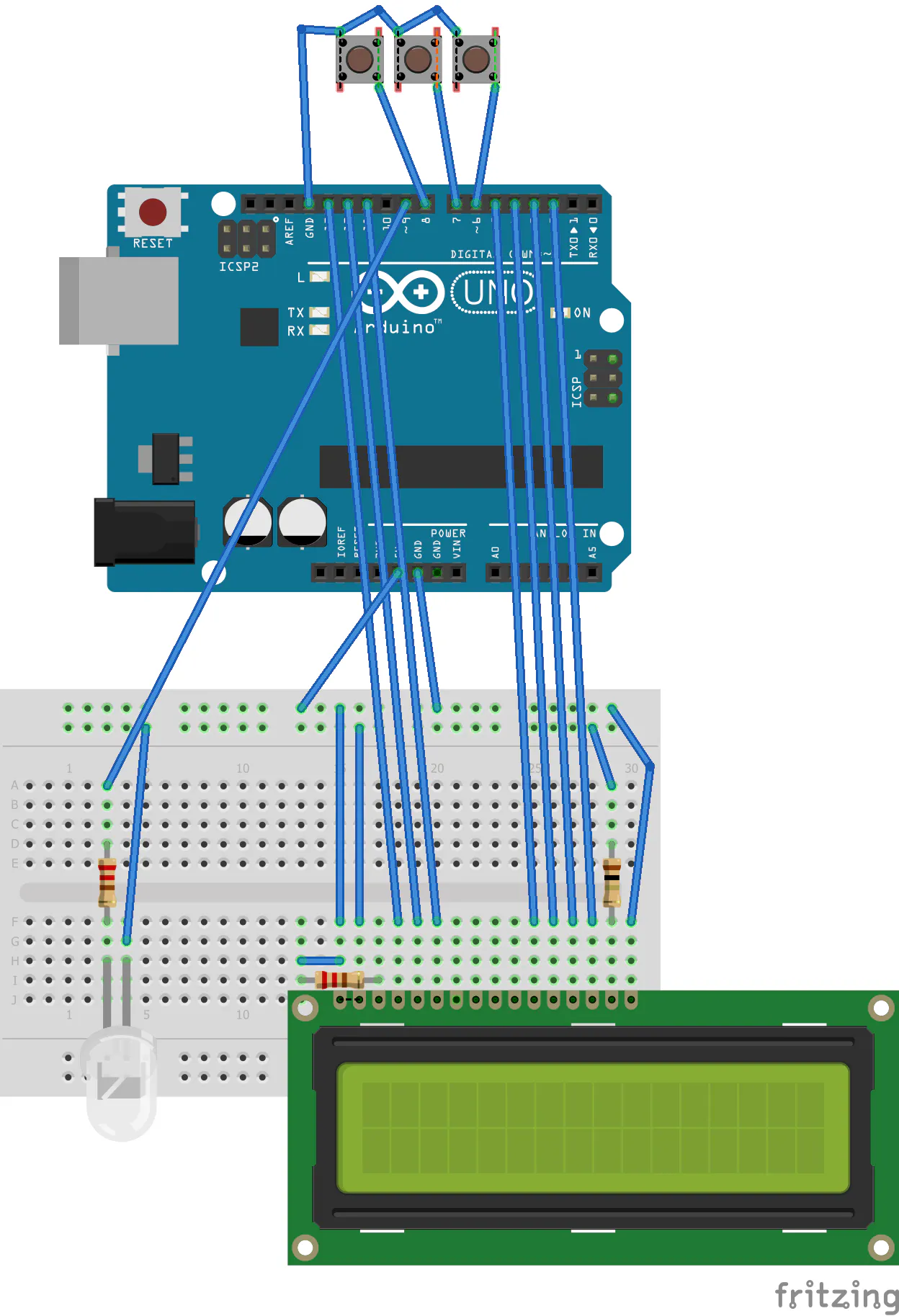## Code

Arduino
The Arduino IDE code to run the Arduino Uno
```#include <LiquidCrystal.h>
LiquidCrystal lcd(12, 11, 10, 5, 4, 3, 2); // ARDUINO UNO
//LiquidCrystal lcd(3, 2, 1, 7, 8, 9, 10); // ATTINY84
byte buttons[] = {6,7,8}; //10 for additional pin
const byte nrButtons = 3; // change to 4 if additional button added
};

int t;
byte pressedButton, currentPos,currentPosParent, possiblePos, possiblePosCount, possiblePosScroll = 0;
String parent = "";

int brightness;
int LCDtoggle = 0;
unsigned long timer = (millis() / 10);
int ledMode = 0;

possiblePosCount = 0;
while (possiblePosCount == 0) {

for (t = 1; t < menusize;t++) {
possiblePos[possiblePosCount]  =  t;
possiblePosCount = possiblePosCount + 1;
}
}

//find the current parent for the current menu
currentPosParent = 0;
for (t = 0; t < menusize; t++) {
if (parent == menu[t]) {currentPosParent = t;}
}

// reached the end of the Menu line
if (possiblePosCount == 0) {
switch (currentPos) {
case 2:
case 3:
case 4:
case 5://Choose between 2,3,4,5
for (t = 2; t<6; t++) {
}
}
break;
case 6: //Toggle
} else {
}

break;
}

//Set Variables
switch (currentPos) {
case 2:
ledMode = 0;
break;
case 3:
ledMode = 1;
break;
case 4:
brightness = 128;
ledMode = 2;
break;
case 5:
brightness = 0;
ledMode = 3;
break;
case 6:
LCDtoggle = abs(LCDtoggle - 1);
digitalWrite(13,LCDtoggle);
break;
}

// go to the parent
currentPos = currentPosParent;
}
}
lcd.clear();

}

// Look for a button press
byte checkButtonPress() {
byte bP = 0;
byte rBp = 0;
for (t = 0; t<nrButtons;t++) {
if (digitalRead(buttons[t]) == 0) {bP = (t + 1);}
}
rBp = bP;
while (bP != 0) { // wait while the button is still down
bP = 0;
for (t = 0; t<nrButtons;t++) {
if (digitalRead(buttons[t]) == 0) {bP = (t + 1);}
}
}
return rBp;
}

void setup() {

lcd.begin(16,2);
lcd.clear();

for (t=0;t<nrButtons;t++) {
pinMode(buttons[t],INPUT_PULLUP);
}

pinMode(13,OUTPUT);
pinMode(9,OUTPUT);

lcd.setCursor(0,0); lcd.print("HELLO");
delay(1000);

}

void loop() {

switch (ledMode) {
case 0:
analogWrite(9, 0);
break;
case 1:
analogWrite(9, 255);
break;
case 3:
if ((millis() / 10) - timer > 30) {
timer = (millis() / 10);
brightness = abs(brightness - 255);
timer = (millis() / 10);
analogWrite(9, brightness);
}
break;
case 2:
if ((millis() / 10) - timer > 3) {
timer = (millis() / 10);
analogWrite(9, brightness);
if (brightness <= 0 || brightness >= 255) {
}
}
break;
}

pressedButton = checkButtonPress();
if (pressedButton !=0) {
switch (pressedButton) {
case 1:
possiblePosScroll = (possiblePosScroll + 1) % possiblePosCount; // Scroll
break;
// If I wanted a 4 button controll of the menu
//case 4:
//  possiblePosScroll = (possiblePosScroll + possiblePosCount - 1) % possiblePosCount; // Scroll
//break;
case 2:
currentPos = possiblePos[possiblePosScroll]; //Okay
break;
case 3:
currentPos = currentPosParent; //Back
possiblePosScroll = 0;
break;
}
}
}
```

### functions

Arduino
Supporting Functions
```String mid(String str, int start, int len) {
int t = 0;
String u = "";
for (t = 0; t < len;t++) {
u = u + str.charAt(t+start-1);
}
return u;
}

int inStrRev(String str,String chr) {
int t = str.length()-1;
int u = 0;
while (t>-1) {
if (str.charAt(t)==chr.charAt(0)) {
u = t+1;t = -1;
}
t = t - 1;
}
return u;
}

int len(String str) {
return str.length();
}
```

## Credits

### Ian Cumming

6 projects • 42 followers
I like stuff, from building rockets to elctronics.